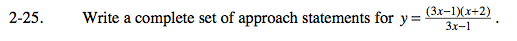### Home > CALC > Chapter Ch2 > Lesson 2.1.2 > Problem2-25

2-25.A complete set of approach statements will include information about what happens when x→∞ and x→−∞. (This will tell us about the location of horizontal asymptotes, if any.) Also, a complete set of approach statements will describe what happens on each side of a hole or vertical asymptote. So the first step will be to determine the location of holes and vertical asymptotes.

$y = \frac{(3x-1)(x+2)}{(3x-1)}=x+2 \text{ with a hole at }x=-2 \text{ and no vertical asymptotes}$

Now that we have determined the location of holes and vertical asymptotes, we can write a complete set of approach statement:

x →−∞, y → __________
x → −2, y → _________
x → ∞, y → __________
x → −2+, y → ________

Use the simplified equation, y = x + 2, to evaluate these approach statements.